# 公交车总迟到？你大概掉进了“等待时间悖论”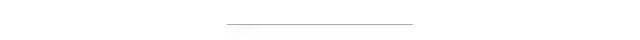import numpy as npN = 1000000  # number of busestau = 10  # average minutes between arrivalsrand = np.random.RandomState(42)  # universal random seedbus_arrival_times = N * tau * np.sort(rand.rand(N))

intervals = np.diff(bus_arrival_times)intervals.mean()

9.9999879601518398

def simulate_wait_times(arrival_times,                       rseed=8675309,  # Jenny's random seed                       n_passengers=1000000):   rand = np.random.RandomState(rseed)      arrival_times = np.asarray(arrival_times)   passenger_times = arrival_times.max() * rand.rand(n_passengers)   # find the index of the next bus for each simulated passenger   i = np.searchsorted(arrival_times, passenger_times, side='right')   return arrival_times[i] - passenger_times

wait_times = simulate_wait_times(bus_arrival_times)wait_times.mean()

10.001584206227317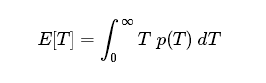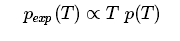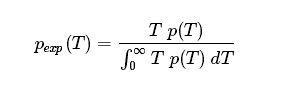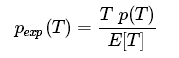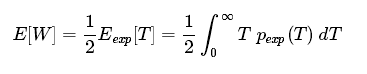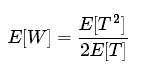### 选择p(T)

%matplotlib inlineimport matplotlib.pyplot as pltplt.style.use('seaborn')plt.hist(intervals, bins=np.arange(80), density=True)plt.axvline(intervals.mean(), color='black', linestyle='dotted')plt.xlabel('Interval between arrivals (minutes)')plt.ylabel('Probability density');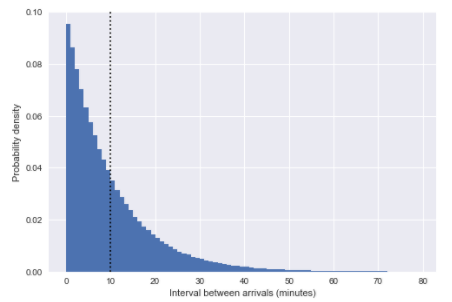from scipy.stats import poisson# count the number of arrivals in 1-hour binsbinsize = 60binned_arrivals = np.bincount((bus_arrival_times // binsize).astype(int))x = np.arange(20)# plot the resultsplt.hist(binned_arrivals, bins=x - 0.5, density=True, alpha=0.5, label='simulation')plt.plot(x, poisson(binsize / tau).pmf(x), 'ok', label='Poisson prediction')plt.xlabel('Number of arrivals per hour')plt.ylabel('frequency')plt.legend();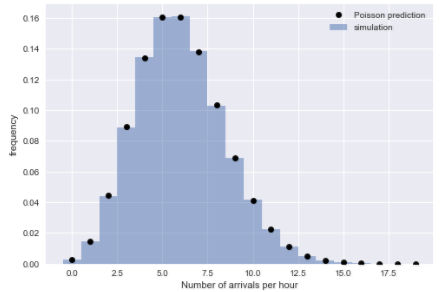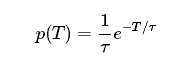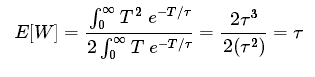import pandas as pddf = pd.read_csv('arrival_times.csv')df = df.dropna(axis=0, how='any')df.head()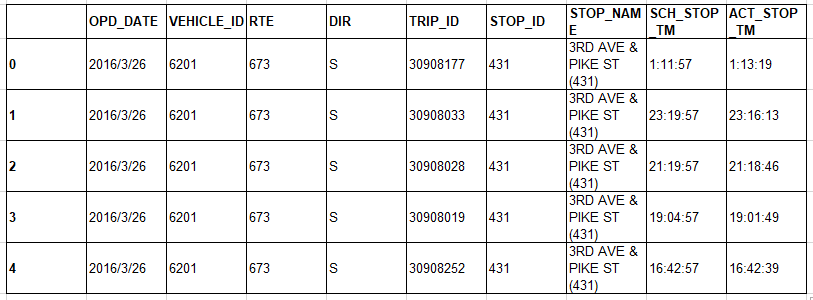### 数据清洗

# combine date and time into a single timestampdf['scheduled'] = pd.to_datetime(df['OPD_DATE'] + ' ' + df['SCH_STOP_TM'])df['actual'] = pd.to_datetime(df['OPD_DATE'] + ' ' + df['ACT_STOP_TM'])# if scheduled & actual span midnight, then the actual day needs to be adjustedminute = np.timedelta64(1, 'm')hour = 60 * minutediff_hrs = (df['actual'] - df['scheduled']) / hourdf.loc[diff_hrs > 20, 'actual'] -= 24 * hourdf.loc[diff_hrs < -20, 'actual'] += 24 * hourdf['minutes_late'] = (df['actual'] - df['scheduled']) / minute# map internal route codes to external route lettersdf['route'] = df['RTE'].replace({673: 'C', 674: 'D', 675: 'E'}).astype('category')df['direction'] = df['DIR'].replace({'N': 'northbound', 'S': 'southbound'}).astype('category')# extract useful columnsdf = df[['route', 'direction', 'scheduled', 'actual', 'minutes_late']].copy()df.head()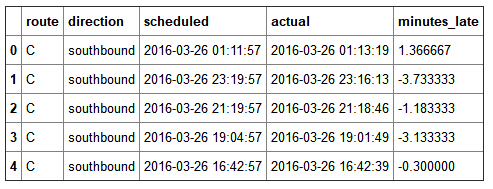### 公交车晚了多少？

import seaborn as snsg = sns.FacetGrid(df, row="direction", col="route")g.map(plt.hist, "minutes_late", bins=np.arange(-10, 20))g.set_titles('{col_name} {row_name}')g.set_axis_labels('minutes late', 'number of buses');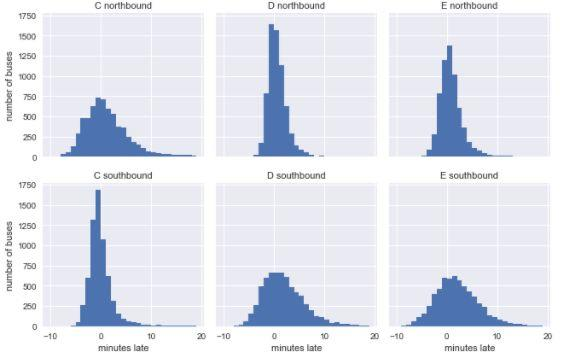### 预定和观察到的到达时间间隔

def compute_headway(scheduled):   minute = np.timedelta64(1, 'm')   return scheduled.sort_values().diff() / minutegrouped = df.groupby(['route', 'direction'])df['actual_interval'] = grouped['actual'].transform(compute_headway)df['scheduled_interval'] = grouped['scheduled'].transform(compute_headway)
g = sns.FacetGrid(df.dropna(), row="direction", col="route")g.map(plt.hist, "actual_interval", bins=np.arange(50) + 0.5)g.set_titles('{col_name} {row_name}')g.set_axis_labels('actual interval (minutes)', 'number of buses');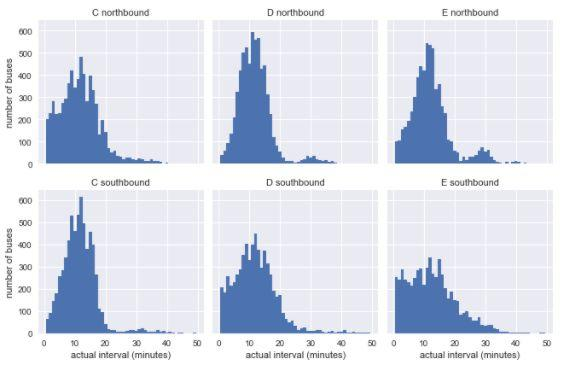g = sns.FacetGrid(df.dropna(), row="direction", col="route")g.map(plt.hist, "scheduled_interval", bins=np.arange(20) - 0.5)g.set_titles('{col_name} {row_name}')g.set_axis_labels('scheduled interval (minutes)', 'frequency');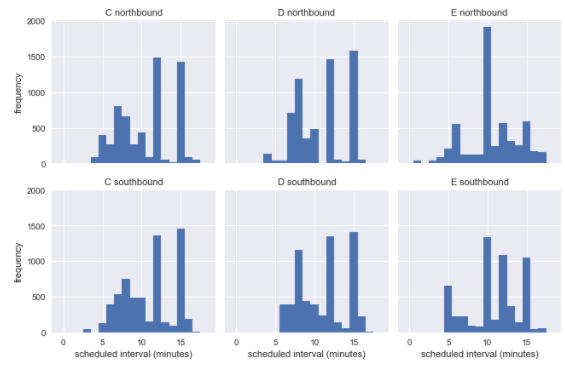def stack_sequence(data):   # first, sort by scheduled time   data = data.sort_values('scheduled')      # re-stack data & recompute relevant quantities   data['scheduled'] = data['scheduled_interval'].cumsum()   data['actual'] = data['scheduled'] + data['minutes_late']   data['actual_interval'] = data['actual'].sort_values().diff()   return datasubset = df[df.scheduled_interval.isin([10, 12, 15])]grouped = subset.groupby(['route', 'direction', 'scheduled_interval'])sequenced = grouped.apply(stack_sequence).reset_index(drop=True)sequenced.head()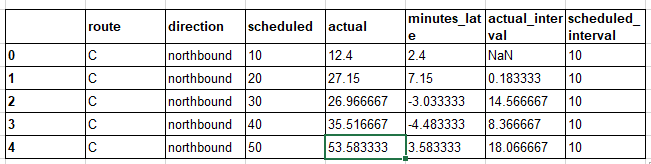for route in ['C', 'D', 'E']:   g = sns.FacetGrid(sequenced.query(f"route == '{route}'"),                     row="direction", col="scheduled_interval")   g.map(plt.hist, "actual_interval", bins=np.arange(40) + 0.5)   g.set_titles('{row_name} ({col_name:.0f} min)')   g.set_axis_labels('actual interval (min)', 'count')   g.fig.set_size_inches(8, 4)   g.fig.suptitle(f'{route} line', y=1.05, fontsize=14)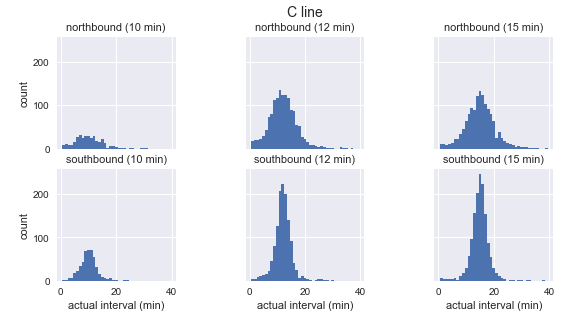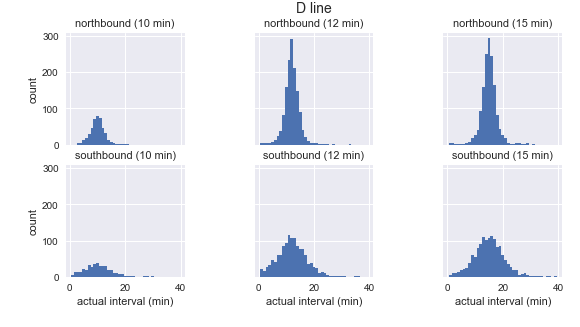grouped = sequenced.groupby(['route', 'direction', 'scheduled_interval'])sims = grouped['actual'].apply(simulate_wait_times)sims.apply(lambda times: "{0:.1f} +/- {1:.1f}".format(times.mean(), times.std()))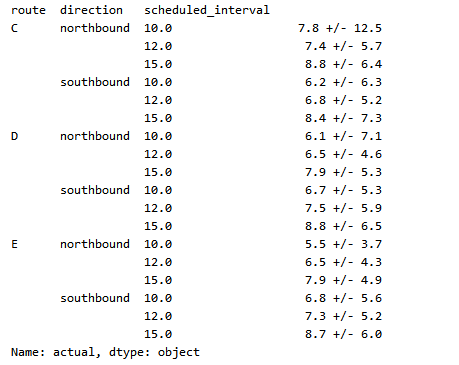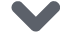点击下方 |  | 了解更多